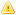Home |Sign in | EnglishHelpWLanguageWLanguage functionsStandard functionsNumeric values management functionsMatrix functionsMatFillPresentationExampleSee alsoFunctions for managing matricesMatAddMatCopyMatCreateMatDeleteMatDeterminantMatErrorMatExistMatFillMatFloatAddMatFloatMultiplyMatInvertMatMultiplyMatNbColumnMatNbLineMatReadMatReadColumnMatReadLineMatStackMatTransposeMatWriteThe matricesThis content has been translated automatically. Click here to view the French version.
 WINDEVWEBDEVWINDEV MobileOthersMatFill (Function) In french: MatRemplit Initializes all the elements found in a matrix of a given size. If the matrix is larger, only the specified elements will be filled.For example:Initialize all the matrix elements with the value 5. This matrix contains 2 rows and 3 columns: ResFill = MatFill("MyMatrix", 5, 2, 3)Initialize the matrix elements from row 1 to row 2 and from column 1 to column 3 with the value 5. Only part of the matrix is initialized: ResFill = MatFill("MyMatrix", 5, 2, 3)Example See additional examples // Fill a matrix containing 2 rows and 3 columns with the number 5ResFill = MatFill("MyMatrix", 5, 2, 3) Syntax = MatFill( , , , ) : BooleanTrue if the fill operation was performed, False otherwise. To find out the name of the error, use the function ErrorInfo with the constant errMessage. : Character string (with quotes)Name of the matrix to fill, defined by MatCreate. : RealValue given to all the matrix elements. : IntegerNumber of rows to fill. : IntegerNumber of columns to fill. Remarks A matrix must exist before it can be filled. To check the matrix existence, use MatExist.Business / UI classification: Neutral codeComponent: wd260mat.dll Minimum version required Version 9 This page is also available for…